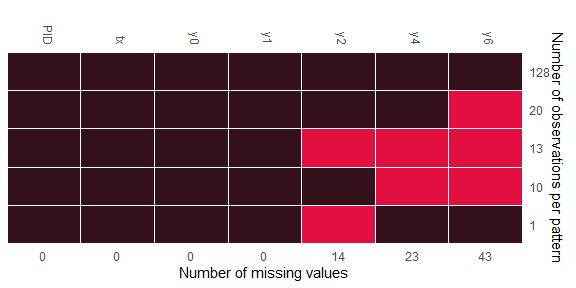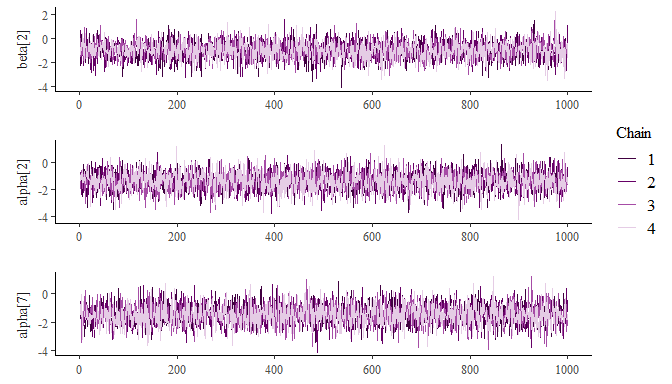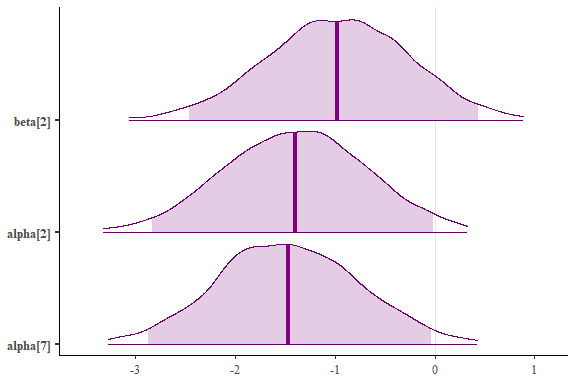### Example: Continuous data imputation through GLM

The example data, which was from an antidepressant clinical trial, can be found in DIA Missing Data webpage. Original data was from an antidepressant clinical trial with four treatments; two doses of an experimental medication, a positive control, and placebo. Hamilton 17-item rating scale for depression (HAMD17) was observed at baseline and its change scores at weeks 1, 2, 4, 6, and 8 ( Goldstein et al. 2004). To mask the real data Week-8 observations were removed. The example data is a sub-sample of the original data: two arms were created; the original placebo arm and a “drug arm” created by randomly selecting patients from the three non-placebo arms.

``````data(antidep)
head(antidep) %>% kbl(align = "c") %>%
kable_classic(full_width = F, html_font = "Cambria") %>%
column_spec(1:2, width = "2cm") %>%
add_header_above(c(" " = 1, " "=1, "Responses at the baseline, week 1, 2, 4, and 6" = 5))
``````
Responses at the baseline, week 1, 2, 4, and 6
PID tx y0 y1 y2 y4 y6
1503 1 32 -11 -12 -13 -15
1507 0 14 -3 0 -5 -9
1509 1 21 -1 -3 -5 -8
1511 0 21 -5 -3 -3 -9
1513 1 19 5 NA NA NA
1514 0 21 2 NA NA NA

Missing pattern is displayed in the following plot:Figure 1. Missing pattern of antidepressant data

The planned statistical method to analyze this endpoint was mixed-effects model with last-observation-carry-forward (LOCF) as the imputation method. In this example, missing values are imputed with GLM models. This is implemented through `family` argument, say, `family = gaussian()` (its default link is `identity`). The same imputation setting is applied for imputing `y2` and `y4`, i.e. argument `models` is set to be `glm_gaussian_identity`. We run the GLM imputation model with an adaptation of 10000 and 2000 iterations for 4 chains. Chains run in parallel, which is set through `doFuture` package:

``````registerDoFuture()
plan(multisession(workers = 4))

an.test = remiod(formula=y6 ~ tx + y0 + y1 + y2 + y4, data=antidep, family = gaussian(),
models = c(y2="glm_gaussian_identity",y4="glm_gaussian_identity"),
n.iter = 100000,  n.chains = 4, n.adapt = 10000, thin=100,
algorithm = "jags", trtvar = 'tx', method="MAR", mess=TRUE, warn=FALSE)

plan(sequential)
``````

The following plots show trace plots and the estimated intervals as shaded areas under the posterior density curves for the parameters of treatment variable `tx` in imputation models:

• beta is the coefficient of tx in imputation model y6 ~ tx + y0 + y1 + y2 + y4;
• alpha is the coefficient of tx in imputation model y4 ~ tx + y0 + y1 + y2;
• alpha is the coefficient of tx in imputation model y2 ~ tx + y0 + y1;

The specified set of parameters can be submitted through argument `subset` with keyword `selected_vars` (alternatively, keyword `selected_parms` can be used):

``````mcsub = get_subset(object = an.test\$mc.mar, subset=c(selected_vars = list("tx")))

color_scheme_set("purple")
mcmc_trace(mcsub, facet_args = list(ncol = 1, strip.position = "left"))
``````Figure 2. Traceplot for coefficients of tx in imputation models

``````mcmc_areas(
mcsub,
prob = 0.95, # 95% intervals
prob_outer = 0.99,
point_est = "mean"
)
``````Figure3. Intervals under the estimated posterior density curves for coefficients of tx in imputation models

To obtain jump-to-reference analysis, we extract MI data with `method="J2R"`, and pool analysis results with `miAnalyze`:

``````j2r = extract_MIdata(object=an.test, method="J2R", M=1000, minspace=4)
res.j2r = miAnalyze(formula = y6 ~ y0 + tx, data = j2r, family = gaussian())

data.frame(res.j2r\$Est.pool) %>% select(-6) %>%
mutate_if(is.numeric, format, digits=4,nsmall = 0) %>%
kbl(align = "c") %>%
column_spec(1:6, width = "3cm") %>%
kable_classic(full_width = F, html_font = "Cambria")
``````
Estimate SE CI.low CI.up t
(Intercept) 1.0433 1.82704 -2.5376 4.6243 0.5711
y0 -0.3292 0.09697 -0.5192 -0.1391 -3.3945
tx -2.3558 1.04973 -4.4133 -0.2984 -2.2442

### Reference

Wang and Liu. 2022. “Remiod: Reference-Based Controlled Multiple Imputation of Longitudinal Binary and Ordinal Outcomes with Non-Ignorable Missingness.” arXiv 2203.02771.